Wednesday, October 2, 2013

Why do we need clustered index

You've probably heard it many times that you have to have Clustered index on each table in your environment and I've also had.

But, I'm mistrustful regarding everything. So, I want to proof or disproof the concept of Clustered Index Necessity.
(All samples are applicable for on premise SQL Server systems. Windows Azure requires to have Clustered index to allow update a tavle)

Test 1. Looking for Data Size.

Use following script to create and populate a table:

 /* Script #1 */   USE TEMPDB; GO SET NOCOUNT ON GO IF EXISTS (   SELECT TOP 1 1 FROM sys.tables   WHERE object_id = OBJECT_ID('tbl_Test_Table_A') ) DROP TABLE tbl_Test_Table_A; GO CREATE TABLE tbl_Test_Table_A(RID int IDENTITY(1,1), F1 char(1), F2 char(1)) GO INSERT INTO tbl_Test_Table_A(F1,F2) VALUES ('0','J'),('1','I'),('2','H'), ('3','G'),('4','F'),('5','E'), ('6','D'),('7','C'),('8','B'),('9','A') GO 53

Now will look at the table size before and after Clustered Index

 /* Script #2 */ SELECT OBJECT_NAME(i.OBJECT_ID) AS TableName, i.name AS TableIndexName, ps.index_id, ps.index_type_desc, ps.index_depth, ps.index_level, ps.page_count, ps.record_count, ps.avg_record_size_in_bytes FROM sys.dm_db_index_physical_stats(DB_ID(), OBJECT_ID('tbl_Test_Table_A'), NULL, NULL, 'DETAILED') ps INNER JOIN sys.indexes i ON i.OBJECT_ID = ps.OBJECT_ID AND i.index_id = ps.index_id; GO CREATE CLUSTERED INDEX CLIX_Test_Table_A ON tbl_Test_Table_A(RID); GO SELECT OBJECT_NAME(i.OBJECT_ID) AS TableName, i.name AS TableIndexName, ps.index_id, ps.index_type_desc, ps.index_depth, ps.index_level, ps.page_count, ps.record_count, ps.avg_record_size_in_bytes FROM sys.dm_db_index_physical_stats(DB_ID(), OBJECT_ID('tbl_Test_Table_A'), NULL, NULL, 'DETAILED') ps INNER JOIN sys.indexes i ON i.OBJECT_ID = ps.OBJECT_ID AND i.index_id = ps.index_id; GO

Here is a result of that query: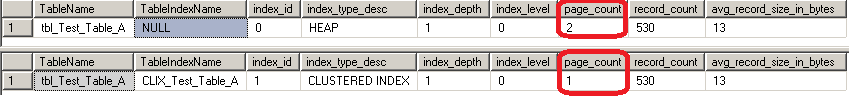As you can see, the number of used pages has decreased.
That means Clustered Index is more compact than a HEAP.

I've looked at the page structure of the table and discovered that HEAP can't handle more than 520 records of that type on a page. It still has free space for extra records, but does not use it.

Were these records compressed by clustered index?  Lets check it.

I will drop clustered index and check table size again:
 /* Script #3 */ SELECT OBJECT_NAME(i.OBJECT_ID) AS TableName, i.name AS TableIndexName, ps.index_id, ps.index_type_desc, ps.index_depth, ps.index_level, ps.page_count, ps.record_count, ps.avg_record_size_in_bytes FROM sys.dm_db_index_physical_stats(DB_ID(), OBJECT_ID('tbl_Test_Table_A'), NULL, NULL, 'DETAILED') ps INNER JOIN sys.indexes i ON i.OBJECT_ID = ps.OBJECT_ID AND i.index_id = ps.index_id; GO DROP INDEX [CLIX_Test_Table_A] ON [dbo].[tbl_Test_Table_A] GO SELECT OBJECT_NAME(i.OBJECT_ID) AS TableName, i.name AS TableIndexName, ps.index_id, ps.index_type_desc, ps.index_depth, ps.index_level, ps.page_count, ps.record_count, ps.avg_record_size_in_bytes FROM sys.dm_db_index_physical_stats(DB_ID(), OBJECT_ID('tbl_Test_Table_A'), NULL, NULL, 'DETAILED') ps INNER JOIN sys.indexes i ON i.OBJECT_ID = ps.OBJECT_ID AND i.index_id = ps.index_id; GO

Here is a result: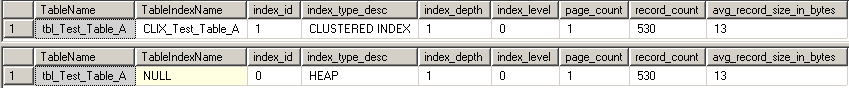As you can see, now 530 records perfectly fit into one page.

Right now I do not have an explanation for that phenomena and might come back to it later.

Test 2. Looking for Index Size.

Run following script to re-create and populate a table by bigger number of records (50K).
That script also creates non-clustered index on column "F2".
 /* Script #4 */   USE TEMPDB GO SET NOCOUNT ON GO IF EXISTS (   SELECT TOP 1 1 FROM sys.tables   WHERE object_id = OBJECT_ID('tbl_Test_Table_A') ) DROP TABLE tbl_Test_Table_A GO CREATE TABLE tbl_Test_Table_A(RID int IDENTITY(1,1), F1 char(1), F2 char(1)) GO CREATE INDEX IX_Test_Table_A ON tbl_Test_Table_A(F2); GO INSERT INTO tbl_Test_Table_A(F1,F2) VALUES ('0','J'),('1','I'),('2','H'), ('3','G'),('4','F'),('5','E'), ('6','D'),('7','C'),('8','B'),('9','A') GO 5000

After that script I run the script #2 and here are it's results: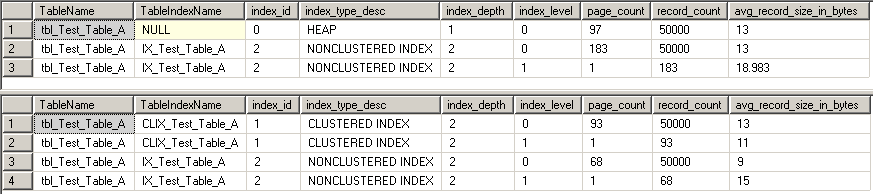As you can see, clustered index added one extra page on a tree level 1, but reduced number of data pages from 97 to 93. So, total reduction of space used by the data is about 3% - not a big deal.

However, number of pages used by non-clustered index dropped significantly..
So, just having clustered index on a table reduces sizes of all other non clustered indexes by up to 60%.

Test 3. In the first two tests I looked mostly at advantages of clustered indexes. Now I want to show disadvantage of HEAP structure.

Lets recreate a table and non-clustered index

 /* Script #5 */   USE TEMPDB GO SET NOCOUNT ON GO IF EXISTS (   SELECT TOP 1 1 FROM sys.tables   WHERE object_id = OBJECT_ID('tbl_Test_Table_A') ) DROP TABLE tbl_Test_Table_A GO CREATE TABLE tbl_Test_Table_A( RID int IDENTITY(1,1),  F1 char(1),  F2 varchar(7000)) GO INSERT INTO tbl_Test_Table_A(F1,F2) VALUES ('0','J'),('1','I'),('2','H'), ('3','G'),('4','F'),('5','E'), ('6','D'),('7','C'),('8','B'),('9','A') GO 52 GO CREATE INDEX IX_Test_Table_A ON tbl_Test_Table_A(F1); GO
*Notice that field F2 now is a varchar(7000).

Now I will update only one record by increasing the size of data to 7000 characters.
 /* Script #6 */ SELECT OBJECT_NAME(i.OBJECT_ID) AS TableName,   i.name AS TableIndexName,   ps.index_id,   ps.index_type_desc,   ps.index_depth,   ps.index_level,   ps.page_count,   ps.record_count,   ps.avg_record_size_in_bytes FROM sys.dm_db_index_physical_stats(DB_ID(),   OBJECT_ID('tbl_Test_Table_A'),   NULL, NULL, 'DETAILED') ps INNER JOIN sys.indexes i   ON i.OBJECT_ID = ps.OBJECT_ID   AND i.index_id = ps.index_id; GO UPDATE tbl_Test_Table_A SET F1 = 'Y', F2 = REPLICATE('Z',7000) WHERE RID = 1 GO SELECT OBJECT_NAME(i.OBJECT_ID) AS TableName,   i.name AS TableIndexName,   ps.index_id,   ps.index_type_desc,   ps.index_depth,   ps.index_level,   ps.page_count,   ps.record_count,   ps.avg_record_size_in_bytes FROM sys.dm_db_index_physical_stats(DB_ID(),   OBJECT_ID('tbl_Test_Table_A'),   NULL, NULL, 'DETAILED') ps INNER JOIN sys.indexes i   ON i.OBJECT_ID = ps.OBJECT_ID   AND i.index_id = ps.index_id; GO

Here is a result of that script: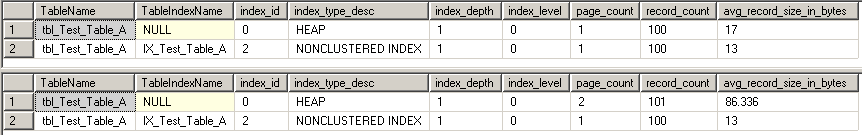As you can see, new page has been created, that is normal.
What is unexpected that number of records in the table has increased by one.

Now I will investigate what was happened.
Run following script to determine Page ID of index page

 DBCC IND('Tempdb','tbl_Test_Table_A',2)
Here is a result. Our Index page Id is 339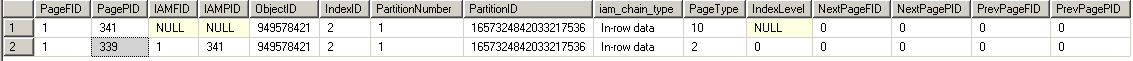Now we will look at records within that table
 DBCC TRACEON(3604) GO DBCC PAGE('Tempdb',1,339,2)

At first I got a header:
 ```PAGE: (1:339) BUFFER: BUF @0x0000000080749F00 bpage = 0x00000000286D8000 bhash = 0x0000000000000000 bpageno = (1:339) bdbid = 2 breferences = 0 bcputicks = 0 bsampleCount = 0 bUse1 = 47223 bstat = 0x10b blog = 0xccdcccdc bnext = 0x0000000000000000 PAGE HEADER: Page @0x00000000286D8000 m_pageId = (1:339) m_headerVersion = 1 m_type = 2 m_typeFlagBits = 0x0 m_level = 0 m_flagBits = 0x8000 m_objId (AllocUnitId.idObj) = 2731977 m_indexId (AllocUnitId.idInd) = 5120 Metadata: AllocUnitId = 1441152059801403392 Metadata: PartitionId = 1657324842033217536 Metadata: IndexId = 2 Metadata: ObjectId = 949578421 m_prevPage = (0:0) m_nextPage = (0:0) pminlen = 10 m_slotCnt = 100 m_freeCnt = 6596 m_freeData = 1409 m_reservedCnt = 0 m_lsn = (0:119329:0) m_xactReserved = 0 m_xdesId = (0:76179) m_ghostRecCnt = 0 m_tornBits = 0 DB Frag ID = 1 Allocation Status GAM (1:2) = ALLOCATED SGAM (1:3) = ALLOCATED PFS (1:1) = 0x60 MIXED_EXT ALLOCATED 0_PCT_FULL DIFF (1:6) = NOT CHANGED ML (1:7) = NOT MIN_LOGGED ```
Index page has 100 records

Record we need is supposed to be the last on that page because 'Y' > '9'
 OFFSET TABLE: Row - Offset                       99 (0x63) - 1396 (0x574)           98 (0x62) - 1383 (0x567)           97 (0x61) - 1370 (0x55a)
Offset for the last record is 0x574

Here is the dump:
 000000000080A564:   02000016 39510100 00010063 00020000 16595101  ....9Q.....c.....YQ. 000000000080A578:   00000100 00000200 00000053 01000001 00510002  ...........S.....Q..
We got it!
- The whole index record is in bold.
- 'Y' is in red
- Reference page number and slot on that page is in blue.
So, we expect our record to be on page 0x151 (337)

Now will prove it:
 DBCC IND('Tempdb','tbl_Test_Table_A',0)
Here is a result
: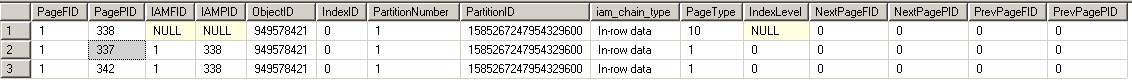And page 337 is there.

 DBCC PAGE('Tempdb',1,337,2)

Lets check what is on that page. Header first:

 ```PAGE: (1:337) BUFFER: BUF @0x000000008074A080 bpage = 0x00000000286D6000 bhash = 0x0000000000000000 bpageno = (1:337) bdbid = 2 breferences = 0 bcputicks = 0 bsampleCount = 0 bUse1 = 48341 bstat = 0x10b blog = 0xcccccccc bnext = 0x0000000000000000 PAGE HEADER: Page @0x00000000286D6000 m_pageId = (1:337) m_headerVersion = 1 m_type = 1 m_typeFlagBits = 0x0 m_level = 0 m_flagBits = 0x8000 m_objId (AllocUnitId.idObj) = 2731352 m_indexId (AllocUnitId.idInd) = 4864 Metadata: AllocUnitId = 1369094465722515456 Metadata: PartitionId = 1585267247954329600 Metadata: IndexId = 0 Metadata: ObjectId = 949578421 m_prevPage = (0:0) m_nextPage = (0:0) pminlen = 9 m_slotCnt = 100 m_freeCnt = 6204 m_freeData = 1796 m_reservedCnt = 8 m_lsn = (543:365:443) m_xactReserved = 8 m_xdesId = (0:76179) m_ghostRecCnt = 0 m_tornBits = 0 DB Frag ID = 1 Allocation Status GAM (1:2) = ALLOCATED SGAM (1:3) = ALLOCATED PFS (1:1) = 0x61 MIXED_EXT ALLOCATED 50_PCT_FULL DIFF (1:6) = NOT CHANGED ML (1:7) = NOT MIN_LOGGED ```
As you can see there are also 100 records on the page

Look for a slot #1 (which is actually 0x00)
 2 (0x2) - 130 (0x82)               1 (0x1) - 113 (0x71)               0 (0x0) - 96 (0x60)
It is pointed to the first record on the page with offset 0x60
Here is the record:
 000000000060A050:   00000000 00000000 00000000 00000000 04560100  .................V..000000000060A064:   00010000 00030000 01001100 4a300009 00020000  ............J0.    ....
Record type in this case is 04 - which is reference
- Page Address 0x156 (342) - which is the next page
- Slot #1 on that page

Lets look for page 342
 DBCC PAGE('Tempdb',1,342,2)
Page has only one record that starts like this:
 000000000080A050:   00000000 00000000 00000000 00000000 32000900  ................2.    .000000000080A064:   01000000 59030000 02006a1b 749b5a5a 5a5a5a5a  ....Y.....j.t›ZZZZZZ
Record type 32 (referenced record)
- Our 'Y' and all 'Zs' are in red
- Offset 0x1b6a (7018) to the end of the record is in blue.

Now we know that extra record that appears in the data set is not a record, but a reference to a real record.
That demonstrates that not having clustered index can generate unnecessary extra reads and corresponding CPU usage.

Hope I've convinced everybody in the necessary of having clustered index.